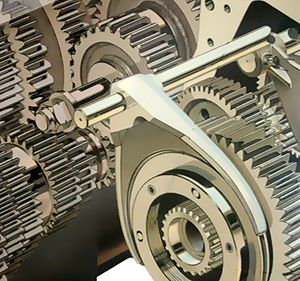# Machine Assembly Round 1

25 Questions | Attempts: 54SettingsAGRONA 2K15INSTRUCTIONS:ALL QUESTIONS ARE MULTIPLE CHOICE QUESTIONONCE ANSWER SELECTED IT CAN'T BE CHANGETIME DURATION:20 MINUTESDON'T CLOSE THE RESULT PAGE,IF CLOSED YOU WILL BE DISQUALIFIEDAFTER COMPLETION INFORM IMMEDIATELY TO THE CO-ORDINATORS   ; &nbs p; &nb sp; &n bsp; ALL THE BEST

• 1.
The bearings of heavy series have capacity __________ over the medium series.
• A.

10 to 20%

• B.

20 to 30%

• C.

30 to 40%

• D.

40 to 50%

• 2.
The size of a gear is usually specified by
• A.

Pressure angle

• B.

Pitch circle diameter

• C.

Circular pitch

• D.

Diametral pitch

• 3.
The backlash for spur gears depends upon
• A.

Module

• B.

Pitch line velocity

• C.

Tooth profile

• D.

Both (a) and (b)

• 4.
The rolling contact bearings are known as
• A.

Thick lubricated bearings

• B.

Plastic bearings

• C.

Thin lubricated bearings

• D.

Antifriction bearings

• 5.
Tapered roller bearings can take
• A.

• B.

• C.

Both radial and axial loads and the ratio of these being less than unity

• D.

Both radial and axial loads and the ratio of these being greater than unity

• 6.
In order to remove internal stresses produced by hardening the steel, the process usually adopted is
• A.

Tempering

• B.

Annealing

• C.

Normalising

• D.

Spheroidising

• 7.
The rivet head used for boiler plate riveting is usually
• A.

• B.

• C.

• D.

• 8.
A localised compressive stress at the area of contact between two members is known as
• A.

Tensile stress

• B.

Bending stress

• C.

Bearing stress

• D.

Shear stress

• 9.
The metal suitable for bearings subjected to heavy loads is
• A.

Silicon bronze

• B.

White metal

• C.

Monel metal

• D.

Phosphor bronze

• 10.
An universal coupling is used to connect two shafts
• A.

Which are perfectly aligned

• B.

Which are not in exact alignment

• C.

Have lateral misalignment

• D.

Whose axes intersect at a small angle\

• 11.
When the shaft rotates in anticlockwise direction at slow speed in a bearing, it will
• A.

Have contact at the bottom most of the bearing

• B.

Move towards right of the bearing making the metal to metal contact

• C.

Move towards right of the bearing making no metal to metal contact

• D.

Move towards left of the bearing making metal to metal contact

• 12.
A taper key which fits half in the key way of the hub and half in the key way of shaft, is known as
• A.

Sunk key

• B.

• C.

• D.

Tangent key

• 13.
When bevel gears having equal teeth and equal pitch angles connect two shafts whose axes intersect at right angle, then they are known as
• A.

Angular bevel gears

• B.

Crown bevel gears

• C.

Internal bevel gears

• D.

Mitre gears

• 14.
In case of pressure vessels having closed ends, the fluid pressure induces
• A.

Longitudinal stress

• B.

Circumferential stress

• C.

Shear stress

• D.

None of these

• 15.
A machine part subjected to __________ is called a strut.
• A.

An axial tensile force

• B.

An axial compressive force

• C.

A tangential force

• D.

Any one of these

• 16.
• A.

Higher

• B.

Lower

• C.

Same

• D.

Option 4

• 17.
Which of the following property is desirable in parts subjected to shock and impact loads?
• A.

Strength

• B.

Stiffness

• C.

Brittleness

• D.

Toughness

• 18.
Pitch point of a cam is
• A.

A point on the pitch curve having minimum pressure angle

• B.

A point on the pitch curve having maximum pressure angle

• C.

Any point on the pitch curve

• D.

Any point on the pitch circle

• 19.
A screw is specified by its
• A.

Major diameter

• B.

Minor diameter

• C.

Pitch diameter

• D.

Pitch

• 20.
Antifriction bearings are
• A.

Thick lubricated bearings

• B.

Plastic bearings

• C.

Thin lubricated bearings

• D.

Ball and roller bearings

• 21.
A special case of ductility which permits materials to be rolled or hammered into thin sheets, is called
• A.

Plasticity

• B.

Elasticity

• C.

Ductility

• D.

Malleability

• 22.
Which of the following welded joint is designed for shear strength ?
• A.

Transverse fillet welded joint

• B.

Parallel fillet welded joint

• C.

Butt welded joint

• D.

All of these

• 23.
The material commonly used for crane hooks is
• A.

Cast iron

• B.

Wrought iron

• C.

Mild steel

• D.

Aluminium

• 24.
In a steam engine, the valve rod is connected to an eccentric rod by means of
• A.

Cotter joint

• B.

Knuckle joint

• C.

Universal joint

• D.

Flange coupling

• 25.
Which of the following formula is used in designing a connecting rod ?
• A.

Euler's formula

• B.

Rankine's formula

• C.

Johnson's straight line formula

• D.

Johnson's parabolic formula

## Related TopicsBack to top
×

Wait!
Here's an interesting quiz for you.